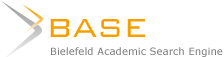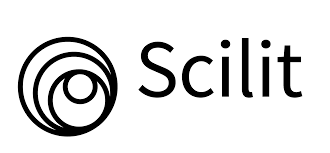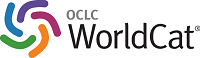### ANALISA PERBANDINGAN KOMPLEKSITAS ALGORITMA SELECTIONSORT DAN INSERTIONSORT

Reza Maulana

#### Abstract

Abstract

Sorting of data is one of the critical operations in data processing. There are various sorting algorithms. However, only some of which are used as the basis for the introduction and understanding of the learning process in an institution. Among the selection sort and insertion sort. Both sorting algorithm that is the focus of discussion on this scientific work. Is the domain on this occasion is a time to try to analyze the speed of the algorithm selection sort and insertion sort, with all the data input in the form of integers in a list or array of data at random intervals between 1,000 and 100,000 elements. Sorting algorithms selection algorithm has a complexity of O (n2 ), the same as the insertion sorting algorithms. Each of these types of algorithms have different levels of effectiveness. The effectiveness of an algorithm can be measured by how the amount of time it takes to run the algorithm. This article will only discuss and analyze the complexity of time for each type of algorithm. The algorithm is written in this paper is an algorithm that is implemented in C ++ programming language. Based on the complexity of the test of time, it can be concluded that the insertionsort algorithm is faster, than the selectionsort algorithm.

#### Full Text:

PDF (Bahasa Indonesia)

#### References

Atrinawati, L. H. (2007). Analisis Kompleksitas Algoritma Untuk Berbagai Macam Metode Pencarian Nilai (Searching) Dan Pengurutan Nilai (Sorting) Pada Tabel. Program Studi Teknik Informatika. Institut Teknologi Bandung.

Joni, I. M. dan Raharjo, B. (2011). Pemrograman C dan Implementasinya. Bandung: Informatika.

Munir, R. (2011). Algoritma dan Pemrograman. Bandung; Informatika.

Suarga. (2012). Algoritma Pemrograman. Yogyakarta: Andi.

Suryani, D. (2013). Perbandingan Metode Bubble Sort dan Insertion Sort Terhadap Efisiensi Memori. Jurnal Teknologi Informasi & Pendidikan. Vol. 6, No. 1.

Tjaru, S. N. B. (2010). Kompleksitas Algoritma Pengurutan Selection Sort dan Insertion Sort. Program Studi Teknik Informatika. Institut Teknologi Bandung.

Yahya, S. Y. (2014). Analisa Perbandingan Algoritma Bubble Sort dan Selection Sort Dengan Metode Perbandingan Eksponensial. Jurnal Pelita Informatika Budi Darma. Vol : VI, No : 3.

Wahyudi, E. N. (2007). Algoritma dan Pemrograman Format Laporan dengan Pascal. Jurnal Teknologi Informasi DINAMIK. Volume XII, No. 1.

DOI: https://doi.org/10.31294/ji.v3i2.810

### Refbacks

• There are currently no refbacks.

### Index by:######### Jl. Kramat Raya No.98, Kwitang, Kec. Senen, Kota Jakarta Pusat, DKI Jakarta 10450